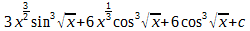# Integration By Parts Practice SheetIntegration is the important part of class XII mathematics.The weightage of Integration in Class XII board exams is about 20 marks.There are so many techniques to find integration of a function.Here we are providing introduction of one technique i.e. Integration by parts with some practice questions.For Integration formulae you can visit our previous post -integration formulae

Integration by Parts is a special method of integration that is often useful when two functions are multiplied together, but is also helpful in other ways.You will see plenty of examples soon, but first let us see the rule:
u v dx = uv dx −u' (v dx) dx
• u is the function u(x)
• v is the function v(x)
Some Questions for practice are given below.....
1.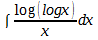2.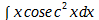3.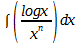4.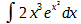5.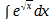6.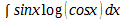7.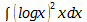8.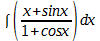9.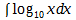10.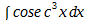11.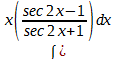12.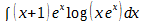13.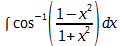14.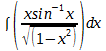15.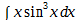16.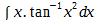17.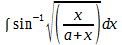18.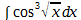1.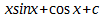2.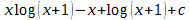3.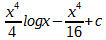4.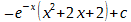5.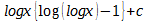6.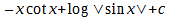7.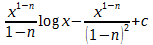8.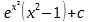9.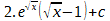10.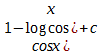11.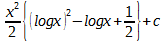12.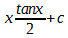13.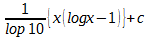14.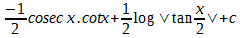15.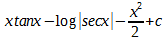16.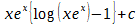17.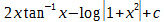18.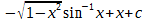19.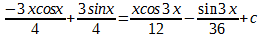20.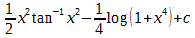21.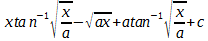22.